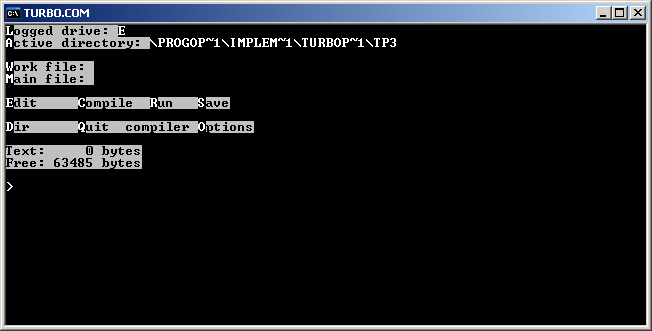# Turbo Pascal 3.0

Version of implementation Turbo Pascal of programming language Pascal

Turbo Pascal 3.0 was the third version of Turbo Pascal series, released on September 17, 1986.

Turbo Pascal 3.0 still looks similar to Turbo Pascal 1.0, though it lists a number of important changes:

• support of EGA color palette and new graphics procedures, including turtle graphics;
• support of overlays;
• support for Binary Coded Decimal (BCD) math;
• lots of new procedures for files processing;
• support of command line parameters processing (if the program is compiled to memory, command line parameters can be set in Options menu).## Examples:

### Hello, World! - Pascal (57):

``````program helloworld;

begin
writeln('Hello, World!');
end.
``````

### Fibonacci numbers - Pascal (58):

This example uses recursive definition of Fibonacci numbers.

``````program fibonacci;

function fib(n:integer): integer;
begin
if (n <= 2) then
fib := 1
else
fib := fib(n-1) + fib(n-2);
end;

var
i:integer;

begin
for i := 1 to 16 do
write(fib(i), ', ');
writeln('...');
end.
``````

### Factorial - Pascal (96):

This example is exactly the same as main recursive example for Pascal implementations, except for that it uses `real` data type to store factorial values. Command `writeln(f:-1:0)` outputs the floating point number `f` with 0 digits after decimal point and left-justifies it.

``````program factorial;

function fact(n: integer): real;
begin
if (n = 0) then
fact := 1
else
fact := n * fact(n - 1);
end;

var
n: integer;

begin
for n := 0 to 16 do
writeln(n, '! = ', fact(n):-1:0);
end.
``````

### Quadratic equation - Pascal (178):

Pascal has built-in complex data type `complex`, but using it is inconvenient in this case, because `writeln` can’t output complex numbers directly, and functions `Re` and `Im` would have to be used. In this example calculations are done in real numbers. Library function `halt` (added in Extended Pascal) exits current block (in later versions it is replaced with `exit`).

``````program Quadratic;

var
A,B,C,D: integer;

begin
write('A = ');
if (A=0) then
begin
halt;
end;
write('B = ');
write('C = ');
D := B*B-4*A*C;
if (D=0) then
begin
writeln('x = ',-B/2.0/A);
halt;
end;
if (D>0) then
begin
writeln('x1 = ',(-B+Sqrt(D))/2.0/A);
writeln('x2 = ',(-B-Sqrt(D))/2.0/A);
end
else
begin
writeln('x1 = (',-B/2.0/A,',',Sqrt(-D)/2.0/A,')');
writeln('x2 = (',-B/2.0/A,',',-Sqrt(-D)/2.0/A,')');
end;
end.
``````